﻿ 关于海南省商品住宅价格模型的分析与预测 Analysis and Forecast of Commodity Housing Price Model in Hainan Province

Statistics and Application
Vol. 08  No. 02 ( 2019 ), Article ID: 29810 , 17 pages
10.12677/SA.2019.82038

Analysis and Forecast of Commodity Housing Price Model in Hainan Province

Wei Teng, Shutong Yue

Shandong University of Science and Technology, Qingdao Shandong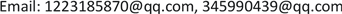Received: Mar. 31st, 2019; accepted: Apr. 15th, 2019; published: Apr. 22nd, 2019ABSTRACT

Based on the data of commodity housing prices in the major cities of Hainan Province and the relevant research literature, this paper makes a quantitative and qualitative analysis of some related factors by using Pearson correlation coefficient in MATLAB software and SPSS software and chi-square test method. Furthermore, principal components are extracted by principal component analysis, regression equation is established, the model is further optimized and tested, and then the mathematical model of commodity housing price in Hainan Province is established, and the rough set theory and grey prediction model are used to find out the biggest correlation index, and forecast the change trend of commodity housing price in Hainan Province. In addition, a VAR model was built to implement the purchase restriction policy Applying and Canceling as virtual variables, autoregressive analysis is carried out with Eviews 8.0. The influence of policy on house price market is discussed quantitatively.

Keywords:Regression Analysis, Housing Price, Principal Component Analysis, Grey Prediction, VAR ModelCopyright © 2019 by authors and Hans Publishers Inc.1. 引言

1) 搜集相关数据，找出决定海南省主要城市商品住宅价格的影响因素，进行相关定性定量分析，并分析各种因素之间的关系。

2) 假设政府没有出台限购政策，结合问题二中建立的数学模型，按照月份预测2018年6月~2019年5月海南省主要城市商品住宅价格。

3) 若考虑2018年4月22日限购政策出台，重新建立数学模型，按照月份预测2018年6月~2019年5月海南省主要城市商品住宅价格。

2. 问题说明

(I) 符号假定

1) 海口城市：

${x}_{1}$ ：居民人均可支配收入；

${x}_{2}$ ：城市经济发展；

${x}_{3}$ ：旅游业；

$y$ ：商品住宅价格。

2) 三亚城市：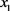：居民人均可支配收入；

${x}_{2}$ ：城市经济发展；

${x}_{3}$ ：城市经济发展；

${x}_{4}$ ：房屋造价；

$y$ ：商品住宅价格。

(II) 模型假设

1) 假定搜集到的数据具有一定的可靠性和真实性；

2) 数据预测时期假定没有出台各种相关政策，受到其他因素的影响都在可控范围内；

3) 海口和三亚两个城市对于海南省有较好的代表性。

(III) 数据预处理

1) 在相关网站上搜集爬取有关数据，并进行统计整理；

2) 使用MATLAB软件，对各种指标的单位进行标准化处理，以消除量纲对模型的影响。

3. 模型的建立与求解

3.1. 问题一分析海南省商品住宅价格的影响因素

3.1.1. 海南省主要城市商品住宅价格变化总体趋势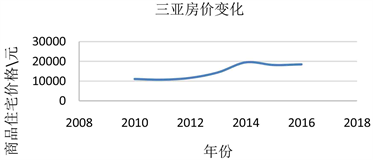Figure 1. Change trend of commercial housing in major cities of Hainan Province

3.1.2. 商品住宅价格影响因素的定性分析

1) 国家宏观政策：

2009年，国务院正式批复《国务院关于推进海南国际旅游岛建设发展的若干意见》，2010年，史上最严的房地产调控政策“新国十条”发布，但海南省受国际旅游岛概念影响，旅游业大幅度发展，游客对房地产需求增大，导致房价大幅上涨。2011年，楼市“三限”、一房一价出台，国家调控力度加大，全国房价继续上涨，海南省房价回落，降为近十年最低价格。2012、2013年，海南省要求清理楼市库存，商品住宅价格下行明显，开发商开始以价换量。

2014年，三亚停建80平以下户型，海口限购松绑，新政出台后，全国楼市普遍迎来成交高峰，但海南成交持续下行。2015、2016年，海南省停建60平以下小户型，中国人民银行降准降息，海南下调住房公积金存贷款利率，海南实施“两个暂停”政策，购房者回归理性，一线城市爆发涨价潮，海南依然以去库存为主，全省库存大幅下降，量价普涨  。

2) 交通发展：

3) 消费者理性预期心理因素：

3.1.3. 商品住宅价格影响因素的定量分析

(I) 海口城市各相关因素变化情况Figure 2. Trend map of various factors in Haikou city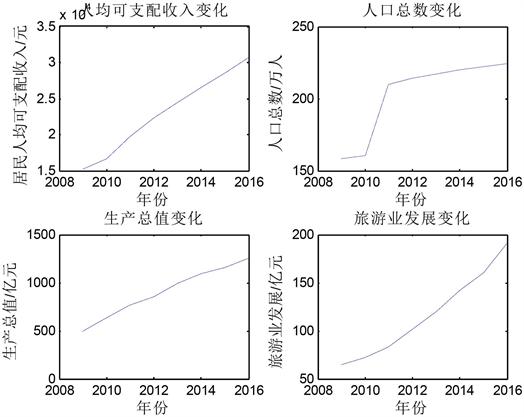Figure 3. Trend diagram of four factors

(II) 三亚城市各相关因素变化情况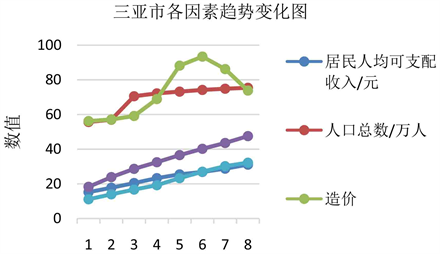Figure 4. Trends of various related factors in Sanya city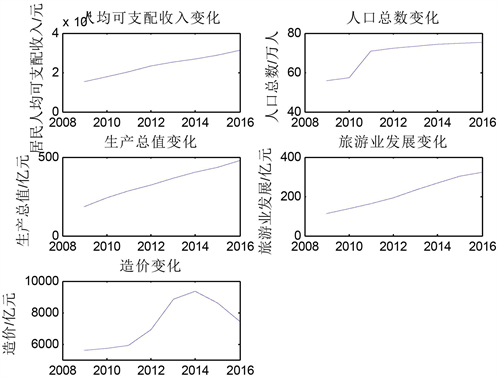Figure 5. Trend Map of various factors in Sanya city

3.2. 构建商品住宅价格数学模型

3.2.1. 初步分析商品住宅价格的线性相关模型

1) 模型建立

$y={\beta }_{0}+{\beta }_{1}{x}_{1}+{\beta }_{2}{x}_{2}+{\beta }_{3}{x}_{3}$

2) 模型求解

3) 残差分析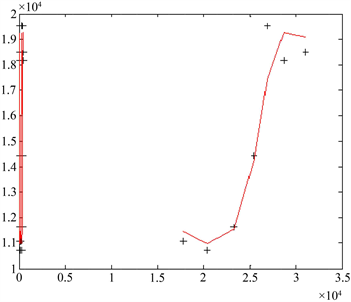Figure 6. Residual plot

3.2.2. 自变量多重共线性的处理

(I) 海口市共线性处理

1) 相关性检验

a) 提取主成分和公因子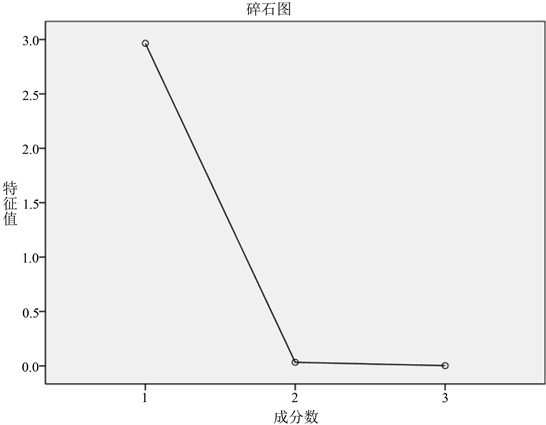Figure 7. Gravel chart

2) 计算正交化单位特征向量

${l}_{i}=\sqrt{{\lambda }_{i}}{e}_{i}\left(i=1,2,3\right)$

3) 建立回归方程并拟合其相关性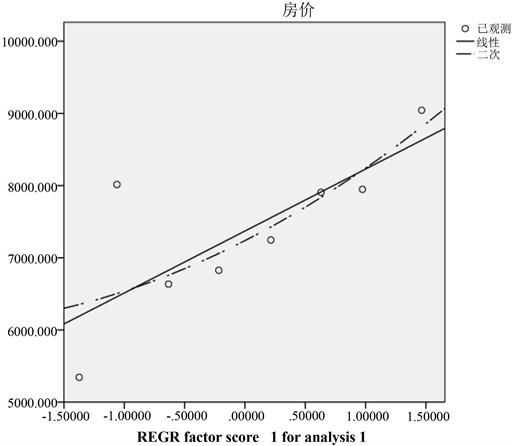Figure 8. Curve fitting graph

$y=\text{0}\text{.766}X$

$y=0.444{x}_{1}+0.443{x}_{2}+0.329{x}_{3}$

(II) 三亚

$y=\text{0}\text{.933}X$

$y=0.48516\left({x}_{1}+{x}_{2}+{x}_{3}\right)+0.40119{x}_{4}$

3.2.3. 模型的最终确定

3.3. 按月分析2018年6月~2019年5月海南省商品住宅房价格

3.3.1. 粗糙集的划分Table 11. Indicators of Hainan province in the past years

$\text{Pos}_c\left\{D\right\}=\left\{2013,2014,2015,2016\right\}$

$\text{Pos}_\left(c-{c}_{2}\right)\left\{D\right\}=\left\{2011,2013,2014\right\}=\text{Pos}_c\left\{D\right\}$

$\text{Pos}_\left(c-{c}_{3}\right)\left\{D\right\}=\left\{2012,2015,2016\right\}=\text{Pos}_c\left\{D\right\}$

3.3.2. 灰色模型GM(1, 1)建立

$\frac{\text{d}{X}^{\left(1\right)}}{\text{d}t}+a{X}^{\left(1\right)}=\mu$ (5.12)

 为待估参数向量，  ，利用最小二乘法求解可得：

$\delta ={\left({B}^{T}B\right)}^{\left(-1\right)}{B}^{T}{Y}_{n}$ (5.13)

${X}^{\left(1\right)}\left(\stackrel{^}{k}+1\right)=\left[{X}^{\left(0\right)}\left(1\right)-\frac{\mu }{a}\right]\cdot {e}^{-ak}+\frac{\mu }{a}\left(k=0,1,2\cdots ,n\right)$ (5.14)

3.3.3. 模型检验校正

$\rho \left(k\right)=1-\left(\frac{1-0.5a}{1+0.5a}\right)\lambda \left(k\right)$

3.3.4. 模型求解

1) 数据检验和预处理

$\lambda \left(k\right)=\frac{{x}^{\left(0\right)}\left(k-1\right)}{{x}^{\left(0\right)}\left(k\right)},k=2,3,\cdots ,n.$

2) 利用matlab编程进行GM(1, 1)建模

$\begin{array}{l}{\delta }_{1}=\left[\begin{array}{l}-0.034\\ 13.185\end{array}\right],{y}_{1}=405.085*{\text{e}}^{0.0342791*t}-384.645\\ {\delta }_{2}=\left[\begin{array}{l}-0.008\\ 2442.56\end{array}\right],{y}_{2}=325800*{\text{e}}^{0.0075673*t}-322772\\ {\delta }_{3}=\left[\begin{array}{l}0.0095\\ 113.909\end{array}\right],{y}_{3}=-11919.2*{\text{e}}^{-0.00945915*t}+12042.2\end{array}$Table 12. Prediction of data based on gray-scale model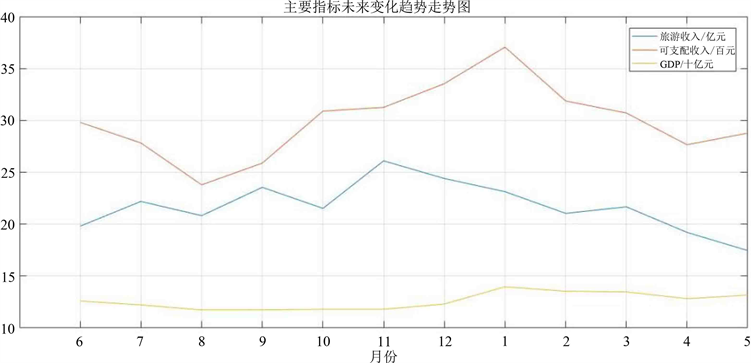Figure 9. Trend of prediction value of each data based on gray-scale model

3) 模型的检验

3.3.5. 模型的代入

1) 海口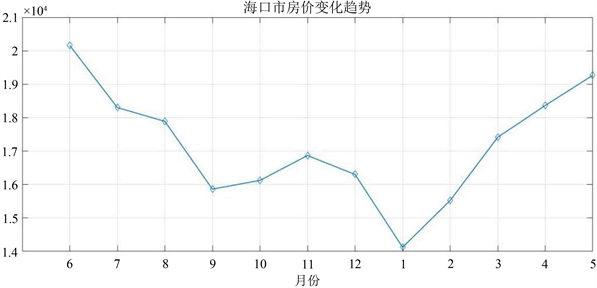Figure 10. Trend of house price forecast in Haikou city

2) 三亚

3.4. 按月分析政策出台后2018年6月~2019年5月海南省商品住宅房价格

3.4.1. 数据预处理及海口市VAR模型的构建

VAR模型对于相互联系的时间序列变量系统是有效的预测模型，对于政策虚拟变量，本问题将运用VAR模型来研究其对商品住宅价格的动态影响。参考文献  ，为减小数据序列的异方差影响，首先对政策虚拟变量取自然对数，得到ln(P)的一个新的时间序列数据。从而建立滞后K期的VAR模型如下：Figure 11. Trend of house price forecast in Sanya city

$\left(\begin{array}{c}\mathrm{ln}{\text{Price}}_{t}\\ \mathrm{ln}{P}_{t}\\ \mathrm{ln}{T}_{t}\\ {\mathrm{lnGDP}}_{t}\end{array}\right)=c+\underset{i=1}{\overset{k}{\sum }}\left(\begin{array}{c}\mathrm{ln}{\text{Price}}_{t-1}\\ \mathrm{ln}{P}_{t-2}\\ \mathrm{ln}{T}_{t-3}\\ {\mathrm{lnGDP}}_{t3}\end{array}\right)+\left(\begin{array}{c}{u}_{1}\\ {u}_{2}\\ {u}_{3}\\ {u}_{4}\end{array}\right)$

3.4.2. 脉冲函数响应分析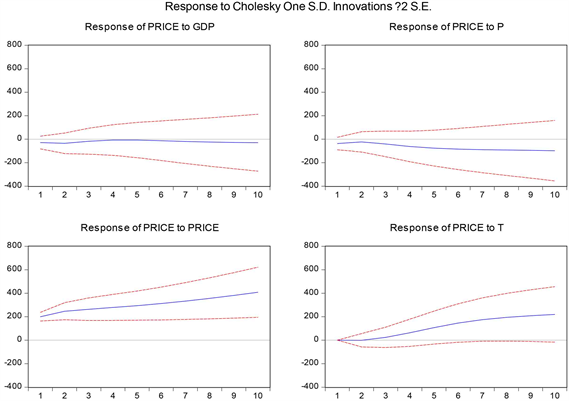Figure 12. Impulse response chart of house price

Impluses只留P (政策虚拟变量)，得到脉冲曲线图13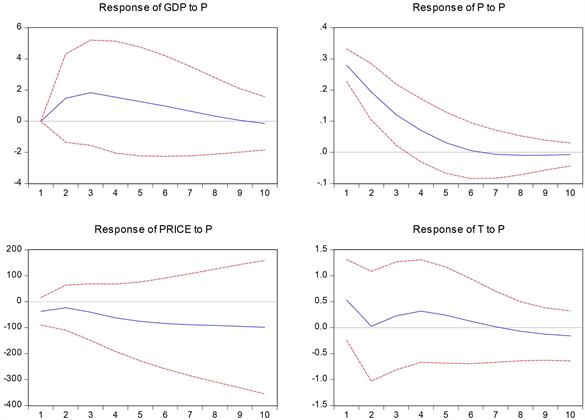Figure 13. Policy dummy variable impulse response graph

3.4.3. 商品住宅价格预测

$\Delta \mathrm{ln}{\text{Price}}_{t}={\left(\begin{array}{c}1.231831\\ 82.31819\\ -0.365957\\ 0.247278\end{array}\right)}^{T}\left(\begin{array}{c}\mathrm{ln}{\text{Price}}_{t-1}\\ \Delta \mathrm{ln}{P}_{t-1}\\ \Delta \mathrm{ln}{T}_{t-1}\\ \Delta \mathrm{ln}{\text{GDP}}_{t-1}\end{array}\right)+{\left(\begin{array}{c}-0.194865\\ -148.1100\\ 10.97197\\ 1.030293\end{array}\right)}^{T}\left(\begin{array}{c}\Delta \mathrm{ln}{\text{Price}}_{t-2}\\ \Delta \mathrm{ln}{P}_{t-2}\\ \Delta \mathrm{ln}{T}_{t-2}\\ \Delta \mathrm{ln}{\text{GDP}}_{t-2}\end{array}\right)-384.6593$

$\Delta \mathrm{ln}{\text{Price}}_{t}={\left(\begin{array}{c}0.917230\\ -649.9250\\ 3.401510\\ 2.948141\end{array}\right)}^{T}\left(\begin{array}{c}\mathrm{ln}{\text{Price}}_{t-1}\\ \Delta \mathrm{ln}{P}_{t-1}\\ \Delta \mathrm{ln}{T}_{t-1}\\ \Delta \mathrm{ln}{\text{GDP}}_{t-1}\end{array}\right)+{\left(\begin{array}{c}0.184801\\ 463.2524\\ 13.73099\\ 0.261242\end{array}\right)}^{T}\left(\begin{array}{c}\Delta \mathrm{ln}{\text{Price}}_{t-2}\\ \Delta \mathrm{ln}{P}_{t-2}\\ \Delta \mathrm{ln}{T}_{t-2}\\ \Delta \mathrm{ln}{\text{GDP}}_{t-2}\end{array}\right)-2143.552$

3.4.4. 模型预测

3.4.5. 结合数据定性分析政策影响

Analysis and Forecast of Commodity Housing Price Model in Hainan Province[J]. 统计学与应用, 2019, 08(02): 341-357. https://doi.org/10.12677/SA.2019.82038

1. 1. 张澜, 周千秋. 市场预期对房地产价格的影响——消费者的理性预期[J]. 中国科技信息, 2006(13): 197-198.

2. 2. 赖一飞, 龙倩倩, 周雅. 城市商品住宅价格合理性实证分析[J]. 科技进步与对策, 2011, 28(13): 152-155.

3. 3. 韩中庚. 数学建模方法及其应用[M]. 北京: 高等教育出版社, 2009.

4. 4. 卓金武. MATLAB在数学建模中的应用[M]. 北京: 北京航空航天大学出版社, 2011.

5. 5. 司守奎, 孙玺菁. 数学建模算法与应用[M]. 北京: 国防工业出版社, 2011.

6. 6. 徐锦, 叶子青. 基于VAR模型的商品房价格影响因素分析[J]. 统计与决策, 2017(11): 95-99.

7. 7. 吴亚楠, 葛鹏, 高聪. 房地产行业的数学建模分析[J]. 数学的实践与认识, 2012, 42(15): 169-179.